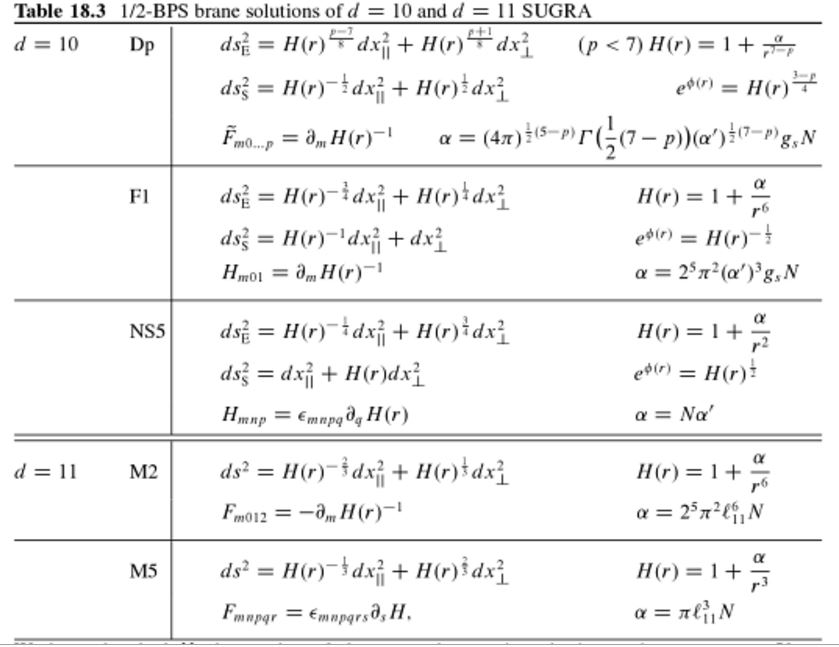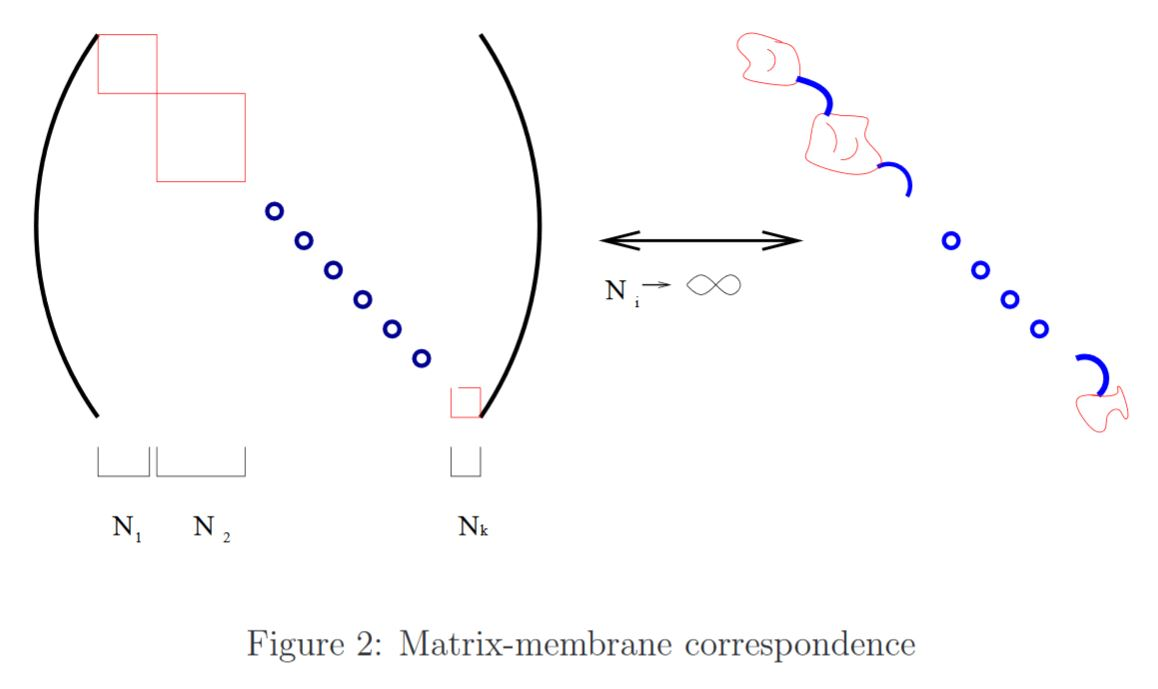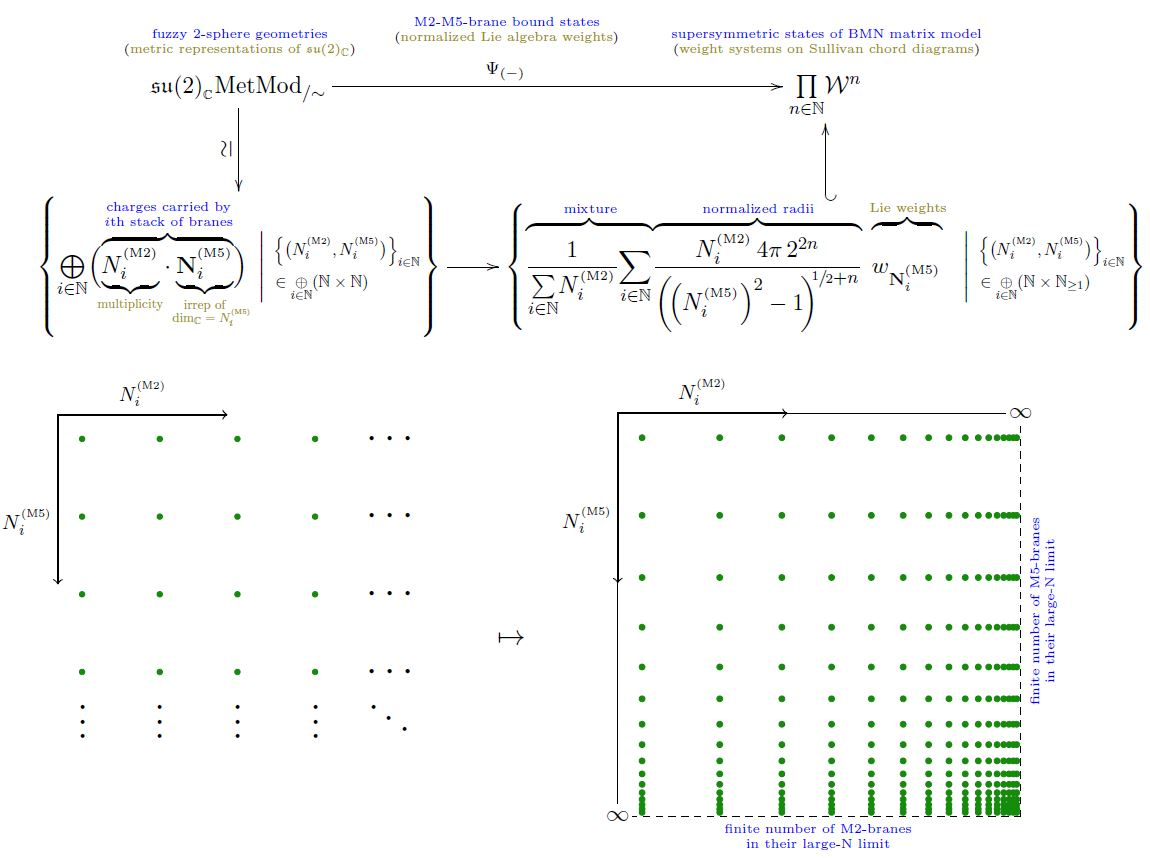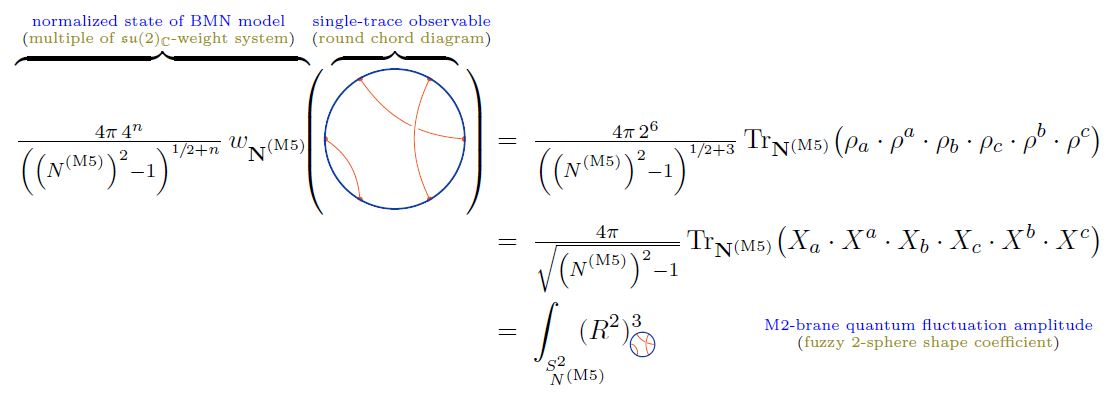Contents

# Contents

## Idea

The theory of 11-dimensional supergravity contains a higher gauge field – the supergravity C-field – that naturally couples to higher electrically charged 2-branes, membranes (Bergshoeff-Sezgin-Townsend 87). By double dimensional reduction, these turn into the superstrings of type IIA string theory (Duff-Howe-Inami-Stelle 87). (See at duality between M-theory and type IIA string theory.)

When in (Witten95) it was argued that the 10-dimensional target space theories of the five types of superstring theories are all limiting cases of one single 11-dimensional target space theory that extends 11-dimensional supergravity (M-theory), it was natural to guess that this supergravity membrane accordingly yields a 3-dimensional sigma-model that reduces in limiting cases to the string sigma-models.

But there were two aspects that make this idea a little subtle, even at this vague level: first, there is no good theory of the quantization of the membrane sigma-model, as opposed to the well understood quantum string. Secondly, that hypothetical “theory extending 11-dimensional supergravity” (“M-theory”) has remained elusive enough that it is not clear in which sense the membrane would relate to it in a way analogous to how the string relates to its target space theories (which is fairly well understood).

Later, with the BFSS matrix model some people gained more confidence in the idea, by identifying the corresponding degrees of freedom in a special case (Nicolai-Helling 98, Dasgupta-Nicolai-Plefka 02). See also at membrane matrix model.

In a more modern perspective, the M2-brane worldvolume theory appears under AdS4-CFT3 duality as a holographic dual of a 4-dimensional Chern-Simons theory. Indeed, its Green-Schwarz action functional is entirely controled by the super-Lie algebra 4-cocycle of super Minkowski spacetime given by the brane scan. This exhibits the M2-brane worldvolume theory as a 3-dimensional higher dimensional WZW model.

## Definition

There are two different incarnations of the M2-brane. On the one hand it is defined as a Green-Schwarz sigma model with target space a spacetime that is a solution to the equations of motion of 11-dimensional supergravity. One would call this the “fundamental” M2 in analogy with the “fundamental string”, if only there were an “M2-perturbation series” which however is essentially ruled out.

On the other hand the M2 also appears as a black brane, hence as a solution to the equations of motion of 11-dimensional supergravity with singularity that looks from outside like a charged 2 dimensional object.

### As a Green-Schwarz sigma model

See at Green-Schwarz sigma model and brane scan.

### As a black brane

#### In the absence of 4-flux

As a black brane solution to the equations of motion of 11-dimensional supergravity, with vanishing C-field flux, the M2 is the spacetime $\mathbb{R}^{2,1} \times (\mathbb{R}^8-\{0\})$ with pseudo-Riemannian metric being

$g = H^{-2/3} g_{\mathbb{R}^{2,1}} + H^{1/3}g_{\mathbb{R}^8-\{0\}}$

where

$H = \alpha + \frac{\beta}{r^6}$

for $(\alpha,\beta) \in \mathbb{R}^2 \setminus \{(0,0)\}$;

and the field strength of the supergravity C-field is

$F = d vol_{\mathbb{R}^{2,1}} \wedge \mathbf{d} H^{-1} \,.$

For $\alpha \beta \neq 0$ this is a 1/2 BPS state of 11d sugra.

In the above coordinates the metric is ill-defined at $r = - \beta^{1/6} \alpha$, but in fact it may be smoothly continued through this point (Duff-Gibbons-Townsend 94, section 3), which is a event horizon. An actual singularity is at $r = 0$.

The near horizon geometry of this spacetime is the Freund-Rubin compactification AdS4$\times$S7. For more on this see at AdS-CFT.(table taken from Blumenhagen-Lüst-Theisen 13, Chapter 18.5)

More generally, one may classify those solutions of 11-dimensional supergravity of the form $AdS_4 \times X_7$ for some closed manifold $X_7$, that are at least 1/2 BPS states. One finds (Medeiros-Figueroa 10) that all these are of the form $AdS_4 \times S^7/G_{ADE}$, where $S^7 / G_{ADE}$ is an orbifold of the 7-sphere (a spherical space form in the smooth case, see there) by a finite subgroup of SU(2) $G_{ADE} \hookrightarrow SU(2)$, i.e. a finite group in the ADE-classification

Dynkin diagram/
Dynkin quiver
dihedron,
Platonic solid
finite subgroups of SO(3)finite subgroups of SU(2)simple Lie group
$A_{n \geq 1}$cyclic group
$\mathbb{Z}_{n+1}$
cyclic group
$\mathbb{Z}_{n+1}$
special unitary group
$SU(n+1)$
A1cyclic group of order 2
$\mathbb{Z}_2$
cyclic group of order 2
$\mathbb{Z}_2$
SU(2)
A2cyclic group of order 3
$\mathbb{Z}_3$
cyclic group of order 3
$\mathbb{Z}_3$
SU(3)
A3
=
D3
cyclic group of order 4
$\mathbb{Z}_4$
cyclic group of order 4
$2 D_2 \simeq \mathbb{Z}_4$
SU(4)
$\simeq$
Spin(6)
D4dihedron on
bigon
Klein four-group
$D_4 \simeq \mathbb{Z}_2 \times \mathbb{Z}_2$
quaternion group
$2 D_4 \simeq$ Q8
SO(8), Spin(8)
D5dihedron on
triangle
dihedral group of order 6
$D_6$
binary dihedral group of order 12
$2 D_6$
SO(10), Spin(10)
D6dihedron on
square
dihedral group of order 8
$D_8$
binary dihedral group of order 16
$2 D_{8}$
SO(12), Spin(12)
$D_{n \geq 4}$dihedron,
hosohedron
dihedral group
$D_{2(n-2)}$
binary dihedral group
$2 D_{2(n-2)}$
special orthogonal group, spin group
$SO(2n)$, $Spin(2n)$
$E_6$tetrahedrontetrahedral group
$T$
binary tetrahedral group
$2T$
E6
$E_7$cube,
octahedron
octahedral group
$O$
binary octahedral group
$2O$
E7
$E_8$dodecahedron,
icosahedron
icosahedral group
$I$
binary icosahedral group
$2I$
E8

Here for $5 \leq \mathcal{N} \leq 8$-supersymmetry then the action of $G_{ADE}$ on $S^7$ is via the canonical action of $SU(2)$ as in the quaternionic Hopf fibration (Medeiros-Figueroa 10), while for $\mathcal{N} = 4$ then there is an extra twist to the action (MFFGME 09). See the table below.

#### In the presence of 4-flux

In the presence of non-vanishing C-field flux $G_4$, the electric flux density of M2-branes is not $G_7 \coloneqq \star G_4$ alone, but receives corrections, first due to the quadratic C-field self-interaction in D=11 supergravity, but then also due to the shifted C-field flux quantization expected in M-theory:

The 11d supergravity literature states the corrected 7-flux to be the following combination, also known as the Page charge (due to Page 83 (8), Duff-Stelle 91 (43), reviewed e.g. in BLMP 13, p. 21):

(1)$\widetilde G'_7 \;\coloneqq\; G_7 + \tfrac{1}{2} C_3 \wedge G_4 \,,$

where the second term subtracts the electric flux induced by the self-intersection of the field, and also ensures that the full expression is a closed differential form if the naive 11d supergravity equations of motion hold:

$d \widetilde G_7 \;=\; 0 \,.$

But in fact (1) does not quite make general sense, for two reasons:

1. In general $G_4 = 0$ is not an admissible condition and is not the actual vanshing of the C-field, due to the shifted C-field flux quantization.

2. Even if $G_4$ happens to be integrally quantizaed (if $\tfrac{1}{4}p_1$ is integral) the appearance of a globally defined C-field potential $C_3$ in (1),means that the total flux actually does vanish after all.

Charge-quantized $\widetilde G_7$-flux with shifted C-field flux quantization (FSS 19b, Prop. 4.3, FSS 19c, Section 4)

Both of these issues are solved if the C-field is taken to be charge quantized in J-twisted Cohomotopy (Hypothesis H). This gives the corrected formula

(2)$\widetilde G_7 \;\coloneqq\; h^\ast G_7 + \tfrac{1}{2} H_3 \wedge h^\ast \widetilde G_4$

where

1. the expression lives on the homotopy pullback of the Sp(2)-parametrized quaternionic Hopf fibration

$h \coloneqq h_{\mathbb{H}}\sslash Sp(2)$

to spacetime, along the twisted Cohomotopy-cocycle that represents the C-field under Hypothesis H;

2. $\widetilde G_4 \coloneqq h^\ast G_4 + \tfrac{1}{4}h^\ast p_1(\nabla)$ is the integral shifted C-field pulled back to that 3-spherical fibration over spacetime;

3. $d H_3 = h^\ast G_4 - \tfrac{1}{4}h^\ast p_1(\nabla)$ trivializes not the C-field itself, but its pullback, and not absolutely but relative to the background charge implied by shifted C-field flux quantization.

With the corrected 7-flux in twisted Cohomotopy it becomes true that

1. the integral of $G_7$ around the 7-sphere linking a black M2-brane is always integer (FSS 19c, Theorem 4.6);

2. this integer satisfies the C-field tadpole cancellation condition (FSS 19b, Section 4.6).

## Properties

### Quantization via BFSS matrix model

A regularized quantization of the Green-Schwarz sigma-model for the M2-brane yields the BFSS matrix model (Nicolai-Helling 98, Dasgupta-Nicolai-Plefka 02).

In this correspondence, matrix blocks around the diagonal correspond to blobs of membrane, while off-diagonal matrix elements correspond to thin tubes of membrane connecting these blobs.graphics grabbed from Dasgupta-Nicolai-Plefka 02

### Worldvolume theory – BLG and ABJM

The worldvolume QFT of black M2-branes is a 3d superconformal gauge field theory:

$d$$N$superconformal super Lie algebraR-symmetryblack brane worldvolume
superconformal field theory
$\phantom{A}3\phantom{A}$$\phantom{A}2k+1\phantom{A}$$\phantom{A}B(k,2) \simeq$ osp$(2k+1 \vert 4)\phantom{A}$$\phantom{A}SO(2k+1)\phantom{A}$
$\phantom{A}3\phantom{A}$$\phantom{A}2k\phantom{A}$$\phantom{A}D(k,2)\simeq$ osp$(2k \vert 4)\phantom{A}$$\phantom{A}SO(2k)\phantom{A}$M2-brane
D=3 SYM
BLG model
ABJM model
$\phantom{A}4\phantom{A}$$\phantom{A}k+1\phantom{A}$$\phantom{A}A(3,k)\simeq \mathfrak{sl}(4 \vert k+1)\phantom{A}$$\phantom{A}U(k+1)\phantom{A}$D3-brane
D=4 N=4 SYM
D=4 N=2 SYM
D=4 N=1 SYM
$\phantom{A}5\phantom{A}$$\phantom{A}1\phantom{A}$$\phantom{A}F(4)\phantom{A}$$\phantom{A}SO(3)\phantom{A}$D4-brane
D=5 SYM
$\phantom{A}6\phantom{A}$$\phantom{A}k\phantom{A}$$\phantom{A}D(4,k) \simeq$ osp$(8 \vert 2k)\phantom{A}$$\phantom{A}Sp(k)\phantom{A}$M5-brane
D=6 N=(2,0) SCFT
D=6 N=(1,0) SCFT

(Shnider 88, also Nahm 78, see Minwalla 98, section 4.2)

Specifically, worldvolume quantum field theory of M2-branes sitting at ADE singularities (as above) is supposed to be described by ABJM theory and, for the special case of $SU(2)$ gauge group, by the BLG model. See also at gauge enhancement.

$N$ Killing spinors on
spherical space form $S^7/\widehat{G}$
$\phantom{AA}\widehat{G} =$spin-lift of subgroup of
isometry group of 7-sphere
3d superconformal gauge field theory
on back M2-branes
with near horizon geometry $AdS_4 \times S^7/\widehat{G}$
$\phantom{AA}N = 8\phantom{AA}$$\phantom{AA}\mathbb{Z}_2$cyclic group of order 2BLG model
$\phantom{AA}N = 7\phantom{AA}$
$\phantom{AA}N = 6\phantom{AA}$$\phantom{AA}\mathbb{Z}_{k\gt 2}$cyclic groupABJM model
$\phantom{AA}N = 5\phantom{AA}$$\phantom{AA}2 D_{k+2}$
$2 T$, $2 O$, $2 I$
binary dihedral group,
binary tetrahedral group,
binary octahedral group,
binary icosahedral group
$\phantom{AA}N = 4\phantom{AA}$$\phantom{A}2 D_{k+2}$
$2 O$, $2 I$
binary dihedral group,
binary octahedral group,
binary icosahedral group
(HLLLP 08b, Chen-Wu 10)

Under AdS-CFT duality the M2-brane is given by AdS4-CFT3 duality. (Maldacena 97, section 3.2, Klebanov-Torri 10).

### M2/M5 bound states

For M2-M5 brane bound states, i.e. bound states of M2-branes with M5-branes (dyonic M2-branes and giant gravitons), see the references below.

For the type II string theory-version see at NS5-brane the sectoin NS5/D4/D2 bound states.

### M2-M5 brane bound states in the BMN matrix model

There is the suggestion (MSJVR 02, checked in AIST 17a, AIST 17b) that, in the BMN matrix model, supersymmetric M2-M5-brane bound states are identified with isomorphism classes of certain “limit sequences” of longitudinal-light cone-constant $N \times N$-matrix-fields constituting finite-dimensional complex Lie algebra representations of su(2).

Concretely, if

$\underset{ i }{\oplus} \big( N^{(M2)}_i \cdot \mathbf{N}^{(M5)}_i \big) \;\;\in\;\; \mathfrak{su}(2)_{\mathbb{C}}Rep^{fin}$

denotes the representation containing

of the

• $N^{(M5)}_i$-dimensional irrep $\mathbf{N}^{(M5)}_i \in \mathfrak{su}(2)_{\mathbb{C}}Rep$

(for $\{N^{(M2)}_i, N^{(M5)}_i\}_{i} \in (\mathbb{N} \times \mathbb{N})^I$ some finitely indexed set of pairs of natural numbers)

with total dimension

$N \;\coloneqq\; dim \big( \underset{ i }{\oplus} \big( N^{(M2)}_i \cdot \mathbf{N}^{(M5)}_i \big) \big)$

then:

1. a configuration of a finite number of stacks of coincident M5-branes corresponds to a sequence of such representations for which

1. $N^{(M2)}_i \to \infty$ (this being the relevant large N limit)

2. for fixed $N^{(M5)}_i$ (being the number of M5-branes in the $i$th stack)

3. and fixed ratios $N^{(M2)}_i/N$ (being the charge/light-cone momentum carried by the $i$th stack);

2. an M2-brane configuration corresponds to a sequence of such representations for which

1. $N^{(M5)}_i \to \infty$ (this being the relevant large N limit)

2. for fixed $N^{(M2)}_i$ (being the number of M2-brane in the $i$th stack)

3. and fixed ratios $N^{(M5)}_i/N$ (being the charge/light-cone momentum carried by the $i$th stack)

for all $i \in I$.

Hence, by extension, any other sequence of finite-dimensional $\mathfrak{su}(2)$-representations is a kind of mixture of these two cases, interpreted as an M2-M5 brane bound state of sorts.

#### Formalization via weight systems on chord diagrams

To make this precise, let

$\mathfrak{su}(2)_{\mathbb{C}} MetMod_{/\sim} \;\in\; Set$

be the set of isomorphism classes of complex metric Lie representations (hence finite-dimensional representations) of su(2) (hence of the special linear Lie algebra $\mathfrak{sl}(2,C)$) and write

$Span \big( \mathfrak{su}(2)_{\mathbb{C}} MetMod_{/\sim} \big) \;\in\; Vect_{\mathbb{C}}$

Finally, write

$\array{ Span \big( \mathfrak{su}(2)_{\mathbb{C}}MetMod_{/\sim} \big) & \longrightarrow & \big( \mathcal{W}^{{}^{s}} \big)^{deg} \\ c_1 \cdot V_1 + c_2 \cdot V_2 &\mapsto& c_1 \cdot w_{V_1} + c_2 \cdot w_{V_2} }$

for the linear map which sends a formal linear combination or representations to the weight system on Sullivan chord diagrams with $deg \in \mathbb{N}$ chords which is given by tracing in the given representation.

Then a M2-M5-brane bound state as in the traditional discussion above, but now formalized as an su(2)-weight system

$\Psi_{ \left\{ N^{(M2)}_i, N^{(M5)}_i \right\}_{i} } \;\in\; \underset{ deg \in \mathbb{N} }{\prod} \big( \mathcal{W}^{{}^{\mathrm{s}}} \big)^{deg}$

hence a weight system horizontal chord diagrams closed to Sullivan chord diagrams, these now being the multi-trace observables on these) is

(3)$\Psi_{ \left\{ N^{(M2)}_i, N^{(M5)}_i \right\}_{i} } \;\coloneqq\; \tfrac{ 4 \pi \, 2^{2\,deg} }{ \sum_i N^{(M2)}_i } \underset{i}{\sum} \left( N^{(M2)}_i \cdot \tfrac{1}{ \left( \sqrt{ \left( N^{(M5)}_i \right)^2 - 1 } \right)^{1 + 2 deg} } \mathbf{N}^{(M5)}_i \right)$graphics from Sati-Schreiber 19c

Normalization and large $N$ limit. The first power of the square root in (3) reflects the volume measure on the fuzzy 2-sphere (by the formula here), while the power of $2\,deg$ (which is the number of operators in the multi-trace observable evaluating the weight system) gives the normalization (here) of the functions on the fuzzy 2-sphere.

Hence this normalization is such that the single-trace observables among the multi-trace observables, hence those which come from round chord diagrams, coincide on those M2-M5 brane bound states $\Psi_{ \left\{ N^{(M2)}_i, N^{(M5)}_i \right\}_{i} }$ for which $N^{(M2)}_i = \delta_i^{i_0} N^{(M2)}$, hence those which have a single constitutent fuzzy 2-sphere, with the shape observables on single fuzzy 2-spheres discussed here:graphics from Sati-Schreiber 19c

Therefore, with this normalization, the limits $N^{(M2)} \to \infty$ and $N^{(M5)} \to \infty$ of (3) should exist in weight systems. The former trivially so, the latter by the usual convergence ofthe fuzzy 2-sphere to the round 2-sphere in the large N limit.

Notice that the multi trace observables on these states only see the relative radii of the constitutent fuzzy 2-spheres: If $N^{(M2)}_i = \delta_i^{i_0} N^{(M2)}$ then the $N^{(M2)}$-dependence of (3) cancels out, reflecting the fact that then there is only a single constituent 2-sphere of which the observable sees only the radius fluctuations, not the absolute radius (proportional to $N^{(M2)}$).

Table of branes appearing in supergravity/string theory (for classification see at brane scan).

branein supergravitycharged under gauge fieldhas worldvolume theory
black branesupergravityhigher gauge fieldSCFT
D-branetype IIRR-fieldsuper Yang-Mills theory
$(D = 2n)$type IIA$\,$$\,$
D(-2)-brane$\,$$\,$
D0-brane$\,$$\,$BFSS matrix model
D2-brane$\,$$\,$$\,$
D4-brane$\,$$\,$D=5 super Yang-Mills theory with Khovanov homology observables
D6-brane$\,$$\,$D=7 super Yang-Mills theory
D8-brane$\,$$\,$
$(D = 2n+1)$type IIB$\,$$\,$
D(-1)-brane$\,$$\,$$\,$
D1-brane$\,$$\,$2d CFT with BH entropy
D3-brane$\,$$\,$N=4 D=4 super Yang-Mills theory
D5-brane$\,$$\,$$\,$
D7-brane$\,$$\,$$\,$
D9-brane$\,$$\,$$\,$
(p,q)-string$\,$$\,$$\,$
(D25-brane)(bosonic string theory)
NS-branetype I, II, heteroticcircle n-connection$\,$
string$\,$B2-field2d SCFT
NS5-brane$\,$B6-fieldlittle string theory
D-brane for topological string$\,$
A-brane$\,$
B-brane$\,$
M-brane11D SuGra/M-theorycircle n-connection$\,$
M2-brane$\,$C3-fieldABJM theory, BLG model
M5-brane$\,$C6-field6d (2,0)-superconformal QFT
M9-brane/O9-planeheterotic string theory
M-wave
topological M2-branetopological M-theoryC3-field on G2-manifold
topological M5-brane$\,$C6-field on G2-manifold
S-brane
SM2-brane,
membrane instanton
M5-brane instanton
D3-brane instanton
solitons on M5-brane6d (2,0)-superconformal QFT
self-dual stringself-dual B-field
3-brane in 6d

### As a fundamental brane (GS-type $\sigma$-model)

The Green-Schwarz sigma-model-type formulation of the supermembrane in 11d (as in the brane scan) first appears in

The equations of motion of the super membrane are derived via the superembedding approach in

and the Lagrangian density for the super membrane is derived via the superembedding approach in

Discussion from the point of view of Green-Schwarz action functional-∞-Wess-Zumino-Witten theory is in

The double dimensional reduction of the M2-brane to the Green-Schwarz superstring was observed in

The interpretation of the membrane as an object related to string theory via double dimensional reduction, hence as the M2-brane was proposed in

around the time when M-theory became accepted due to

• Paul Howe, Ergin Sezgin, The supermembrane revisited, (arXiv:hep-th/0412245)

• Igor Bandos, Paul Townsend, SDiff Gauge Theory and the M2 Condensate (arXiv:0808.1583)

• M.P. Garcia del Moral, C. Las Heras, P. Leon, J.M. Pena, A. Restuccia, Fluxes, Twisted tori, Monodromy and $U(1)$ Supermembranes (arXiv:2005.06397)

• Pedro D. Alvarez, Pedro García, Maria Pilar Garcia del Moral, Joselen M. Peña, Reginaldo Prado, Spinning solutions for the bosonic M2-brane with $C_{\pm}$ fluxes (arXiv:2104.08927)

### Quantization of the M2-brane sigma-model to a matrix model

The Poisson bracket-formulation of the classical light-cone gauge Hamiltonian for the bosonic relativistic membrane and the corresponding matrix commutator regularization is due to:

On the regularized light-cone gauge quantization of the Green-Schwarz sigma model for the M2-brane on (super) Minkowski spacetime, yielding the BFSS matrix model:

Original articles:

Observation that the spectrum is continuous:

Review:

The generalization to pp-wave spacetimes (leading to the BMN matrix model):

### As a black brane

The black membrane solution of 11-dimensional supergravity was found in

Its regularity across the event horizon is due to

Further discussion of such Freund-Rubin compactifications:

The Horava-Witten-orientifold of the black M2, supposedly yielding the black heterotic string is discussed in

• Zygmunt Lalak, André Lukas, Burt Ovrut, Soliton Solutions of M-theory on an Orbifold, Phys. Lett. B425 (1998) 59-70 (arXiv:hep-th/9709214)

• Ken Kashima, The M2-brane Solution of Heterotic M-theory with the Gauss-Bonnet $R^2$ terms, Prog.Theor.Phys. 105 (2001) 301-321 (arXiv:hep-th/0010286)

Meanwhile AdS-CFT duality was recognized in

where a dual description of the worldvolume theory of M2-brane appears in section 3.2. More on this is in

An account of the history as of 1999 is in

More recent review is in

• Georgios Linardopoulos, chapter 13 of Classical Strings and Membranes in the AdS/CFT Correspondence (pdf, spire)

A detailed discussion of this black brane-realization of the M2-brane and its relation to AdS-CFT is in

The generalization of this to $\geq 1/2$ BPS sugra solutions of the form $AdS_4 \times X_7$ is due to

Discussion of $G7$-C-field flux quantization in the $G_4$-fluxed case:

Review:

### History

Discussion of the history includes

### Decoupled worldvolume theory

On the BLG model/ABJM model for the worldvolume-theory of coincident? M2-branes see there.

Review:

### Dualities

The role of and the relation to duality in string theory of the membrane is discussed in the following articles.

Relation to T-duality is discussed in:

• J.G. Russo, T-duality in M-theory and supermembranes (arXiv:hep-th/9701188)

• M.P. Garcia del Moral, J.M. Pena, A. Restuccia, T-duality Invariance of the Supermembrane (arXiv:1211.2434)

Relation to U-duality is discussed in:

Discussion from the point of view of E11-U-duality and current algebra is in

### M2-M5 bound states

Discussion of M2-M5 brane bound states, i.e. dyonic$\,$black M2-branes (M5-branes wrapped on a 3-manifold, see also at NS5-branes – D2/D4/NS5-bound states):

Further M2/M5-brane bound states to giant gravitons:

• J. M. Camino, A. V. Ramallo, M-Theory Giant Gravitons with C field, Phys. Lett. B525:337-346, 2002 (arXiv:hep-th/0110096)